The function of the print statement
Custom SearchThe function of the PRINT statement is to instruct the computer to output something, either on the terminal or the printer. It can be used in several different ways. One way is to print the value of a single variable.

Example:

Printing the value of a single variable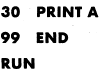This statement will cause the computer to print whatever value it has for the variable A. The output will be the value of A only, the variable name A will not be printed. Let’s assume the value of A in the PRINT statement is 896. When the PRINT statement is executed, the output will be:

896

Note that only the value of variable A was printed, not the variable name.

Now let’s suppose you wanted to print the values of several variables. It could be done this way:

Example:

Printing the values of three variables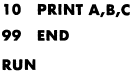Assume the values of A, B, and C are 896, 754, and 969 respectively. The output from the PRINT statement would look like this: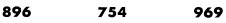Whenever you wish to print several variables with one PRINT statement, you must separate them with either commas or semicolons, as was done in line number 10 of the example. The commas will give you standard spacing. If semicolons had been used, we would have gotten packed spacing. More on the use of the comma and semicolon will be discussed later in the chapter.

Printing the results of a computation is another use of the PRINT statement. Let’s suppose we want to get the average of three scores: 82, 95, and 93. First we must define the problem: how to compute an average. You add up the scores and divide by the number of scores. This problem can be solved by using a PRINT statement like this:

Example:

Print the results of a computation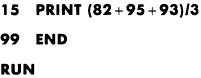We have used a simple mathematical formula in conjunction with the PRINT statement to solve the problem. First the computer will add all the scores, then it will divide by three and print the result. When this statement is executed, the output will be:

90

This output is rather plain and meaningless to anyone other than the person who wrote the program. There is another use of the PRINT statement which will enhance the output from the previous example, printing a message using the PRINT statement. Any message to be printed must be enclosed in quotation marks.

Example:

Printing a message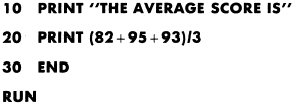The output from these PRINT statements would be: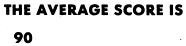You will notice the quotation marks around the message in line 10 causes the message to be printed just as it appears in the program. Using the PRINT statement in this manner adds clarity and meaning to the output.

Now let’s look at another combination of uses of the PRINT statement. Suppose we wanted to print out the individual scores and the average of the scores, with a blank line between them. The following example shows how ‘ this could be done:

Example:

Printing blank lines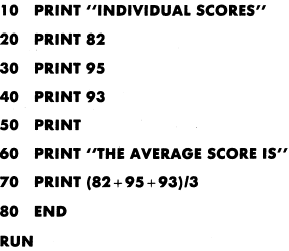Note that line 50 contains a blank PRINT statement. This will cause spacing between lines of output. It can be used to provide clarity and improve readability. When the program is executed, the output will look like this:As seen in this example the blank PRINT statement produced a blank line between the individual scores and the average score. This feature may work differently on some computers.

We could have written the previous example in semicolons to separate messages and numbers. a different manner—using

Example:

Printing messages and numbers on the same line,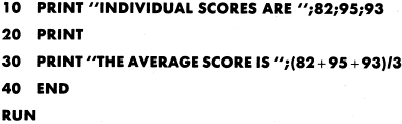The information enclosed in quotation marks will be printed exactly as it appears in the program followed by the numeric data in line 10 and the result of the computation in line 30.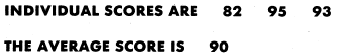The various uses of the PRINT statement have been presented. Let’s take a closer look at the punctuation used in PRINT statements, and how it affects the output. The two primary punctuation marks used in a PRINT state-ment are the comma and semicolon.Integrated Publishing, Inc. - A (SDVOSB) Service Disabled Veteran Owned Small Business